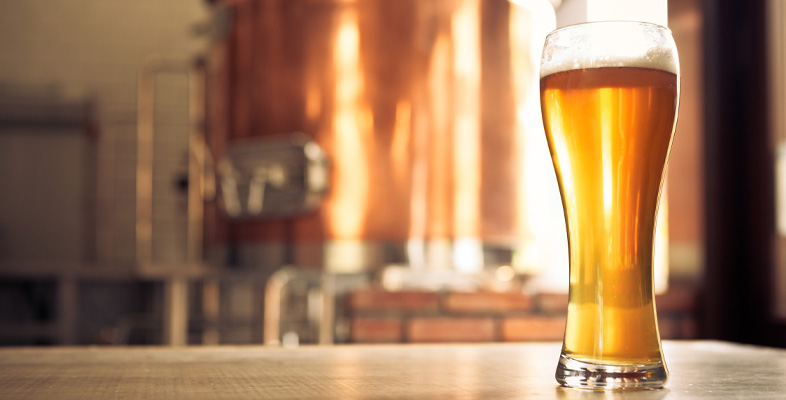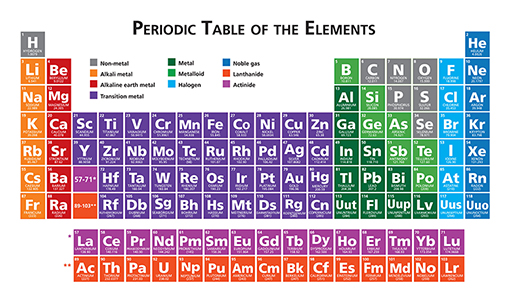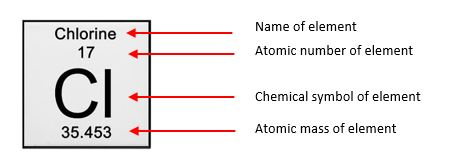Science, Maths & Technology

### Become an OU studentThe science of alcohol

Start this free course now. Just create an account and sign in. Enrol and complete the course for a free statement of participation or digital badge if available.

# 3.1 Relative molecular mass (RMM)

The size of the molecule is a key factor in odorants, which are normally quite small. This means they tend to have a relative molecular mass (RMM) of less than 300.

Atoms of different elements all have different masses, referred to as atomic masses. The atomic mass of a particular element can be located on the periodic table.

The periodic table is a display of all known elements arranged in order of their atomic number. The complete periodic table is illustrated in Figure 3 below.Figure 3 The periodic table, an arrangement of all known chemical elements in order of increasing atomic number

You will learn what is meant by ‘atomic number’ in Week 8. However, in addition to the atomic number, the atomic mass of each element can also be read from its position in the periodic table. For example, chlorine (Cl) has an atomic mass of 35.453, as illustrated in Figure 4.Figure 4 The properties of chlorine from the periodic table
• Water, H2O, comprises two atoms of hydrogen (atomic mass 1.008) and one atom of oxygen (atomic mass 15.999). What is the molecular mass of water?

• The molecular mass of water = (atomic mass of hydrogen x 2) + atomic mass of oxygen.

The molecular mass of water = (2 x 1.008) + 15.999 = 18.015.

At this stage you may be wondering about units, and rightly so, but relative mass is actually a unitless quantity. Why is this? Atoms are very small particles and so their masses are also very small. It is rather awkward to conceive of and work with such small numbers, so a convention has been adopted in which the masses of atoms are related to a standard atomic mass.

The standard chosen is a form of carbon called carbon-12, the mass of which is defined to be exactly 12. So, if you divide the mass of an atom of any element by the mass of a carbon-12 atom, then multiply the result by 12, this gives you its atomic mass relative to carbon-12. Any units of mass will cancel out, and this is why both relative atomic mass (RAM, for an element) and relative molecular mass (RMM, for a compound) are unitless quantities.

• Considering the atom chlorine as an example. Looking back at Figure 3, what is the atomic mass of chlorine?

• The atomic mass of chlorine is 35.453 amu (where amu stands for ‘atomic mass unit’)

To work out the mass of an atom of chlorine relative to carbon -12